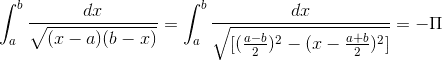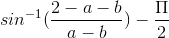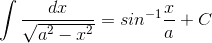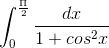# Definite Integral

## Homework StatementI am getting the answer as -(pi)
The answer given is (pi). There might be a sign error. I checked it many times but cannot find my fault. I need some help.

Mark44
Mentor

## Homework StatementThe quantities in the two radicals are equal, so I don't see a problem there. One problem that I see that you might not have addressed is that the integral you started with is improper - the integrand is undefined at both x = a and x = b.
I am getting the answer as -(pi)
The answer given is (pi). There might be a sign error. I checked it many times but cannot find my fault. I need some help.

## The Attempt at a Solution

One problem that I see that you might not have addressed is that the integral you started with is improper - the integrand is undefined at both x = a and x = b.

Then that should lead to an indeterminable integral. Why there is only a difference in sign?

How do I proceed the correct way?

Mark44
Mentor
Then that should lead to an indeterminable integral.
Not necessarily. You need to split the original integral into two integrals, and use limits to evaluate each.
$$\lim_{p \to a^+}\int_p^1 \frac{dx}{\sqrt{(x - a)(b - x)}} + \lim_{q \to b^-}\int_1^q \frac{dx}{\sqrt{(x - a)(b - x)}}$$
Why there is only a difference in sign?
I have no way of knowing, since I don't know what you did.
How do I proceed the correct way?
See above.

Char. Limit
Gold Member
I find it odd that while the integral is indeterminate, the indefinite integral gives you a function that exists and is finite even at x=a and x=b.

Mark44
Mentor
There are lots of functions that are defined everywhere, but whose derivatives fail to exist at one or more points. E.g., y = |x|. If you differentiate the antiderivative, you get the integrand.

jgens
Gold Member
Char. Limit: I'm not sure what you mean by indeterminate, since according to the OP its supposed to converge to $\pi$. But the fact that the anti-derivative exists and is defined at $x=a$ and $x=b$ really shouldn't be all that surprising. Just think about the function defined by $f(x) = \sqrt{x}$. As $x \to 0$ the slope of the tangent line increases without bound, and therefore its derivative isn't defined at 0 even though the function is defined at 0.

Char. Limit
Gold Member
Yes, you're right of course. Don't know what I was thinking there.

$$\lim_{p \to a^+}\int_p^1 \frac{dx}{\sqrt{(x - a)(b - x)}} + \lim_{q \to b^-}\int_1^q \frac{dx}{\sqrt{(x - a)(b - x)}}$$

I am getting the first integral as (after applying limit)Is it right?

Mark44
Mentor
How are you getting the sin-1 part? I used wolfram alfpha, and the indefinite integral involves the inverse tangent function.

When I evaluated the first integral I got -[sqrt(1-a)sqrt(b-1)tan^(-1)((a+b-2)/2sqrt(1-a)sqrt(b-1))] / [sqrt(1-a)(b-a)] + pi/2.

I suspect that the second integral ends up wiping out the first term above + another pi/2.

hunt_mat
Homework Helper
Okay, here is a simple explanation, take the domain of integration a<x<b, then everything will be fine and we're not changing the result.
Note
$$\int_{a}^{b}\frac{dx}{\sqrt{(x-a)(b-x)}}=\int_{a}^{b}\frac{dx}{\sqrt{\left(\frac{b-a}{2}\right)^{2} -\left( x-\frac{a+b}{2}\right)^{2}}}$$
Use the substitution:
$$y=x-\frac{a+b}{2}$$
To obtain the integral:
$$\int_{-\alpha}^{\alpha}\frac{dy}{\sqrt{\alpha^{2}-y^{2}}}$$
Then use the substitution $$y=\alpha\sin z$$ to get the answer quoted. I will leave you to fill in the details and find out what alpha is.

Okay, here is a simple explanation, take the domain of integration a<x<b, then everything will be fine and we're not changing the result.
Note
$$\int_{a}^{b}\frac{dx}{\sqrt{(x-a)(b-x)}}=\int_{a}^{b}\frac{dx}{\sqrt{\left(\frac{b-a}{2}\right)^{2} -\left( x-\frac{a+b}{2}\right)^{2}}}$$
Use the substitution:
$$y=x-\frac{a+b}{2}$$
To obtain the integral:
$$\int_{-\alpha}^{\alpha}\frac{dy}{\sqrt{\alpha^{2}-y^{2}}}$$
Then use the substitution $$y=\alpha\sin z$$ to get the answer quoted. I will leave you to fill in the details and find out what alpha is.

This is much better.
But I see no use of restricting the domain other than our own satisfaction. I mean we don't use that condition anywhere in the solution later.
I found out that there is a change in sign only because of taking {(a-b)^2}/2 as I did instead of {(b-a)^2}/2 as you did.

The formula forIs there any change to be made in this formula such as applying mod. function so that I can use it in both the cases {(a-b)^2}/2 & {(b-a)^2}/2 which are equivalent algebraically but not in calculus?

How are you getting the sin-1 part? I used wolfram alfpha, and the indefinite integral involves the inverse tangent function.

When I evaluated the first integral I got -[sqrt(1-a)sqrt(b-1)tan^(-1)((a+b-2)/2sqrt(1-a)sqrt(b-1))] / [sqrt(1-a)(b-a)] + pi/2.

I suspect that the second integral ends up wiping out the first term above + another pi/2.

Did you miss the root? How can you get an arctan function with a root?
I applied the formula I gave in the previous post and got arcsin.

hunt_mat
Homework Helper
As the integral was from a to b, I made the assumption that b>a, and so the term (b-a).2>0.

Technically what we're doing is taking away what is known as a null set {a,b}, this will have no effect whatsoever on the value of the integral. The signs should all cancel out in the end.

Mat

Mark44
Mentor
Did you miss the root? How can you get an arctan function with a root?
No, I didn't omit the square root. This is what I entered into wolframalpha:
integrate 1/sqrt((x-a)(b-x)) dx

The result was what I posted earlier in this thread.
I applied the formula I gave in the previous post and got arcsin.
It is often the case that integrating by two different techniques gives different antiderivatives, but they can differ at most by a constant.

Last edited:
Mark44
Mentor
As the integral was from a to b, I made the assumption that b>a, and so the term (b-a).2>0.

Technically what we're doing is taking away what is known as a null set {a,b}, this will have no effect whatsoever on the value of the integral. The signs should all cancel out in the end.
At some point you are using the Fundamental Theorem of Calculus to find an antiderivative, which is then evaluated at the two endpoints of the interval. The FTC requires the integrand to be continuous on the closed interval [a, b], which means it must be also defined at all points in the interval, including the two endpoints. This is the reason for the need to work with limits at both endpoints in this problem. The fact that we're dealing with a set of measure zero is irrelevant.

No, I didn't omit the square root. This is what I entered into wolframalpha:
integrate 1/sqrt((x-a)(b-x)) dx

The result was what I posted earlier in this thread.

It is often the case that integrating by two different techniques gives different antiderivatives, but they can differ at most by a constant.

I entered the function there and got it as -

[PLAIN]http://www4b.wolframalpha.com/Calculate/MSP/MSP258319ddgi17bga1e8hh000021dhe85848hb983f?MSPStoreType=image/gif&s=33&w=483&h=62 [Broken]

How did you evaluate the limit? Is it not too complex? Why are the like terms in numerator and denominator not cancelled here in wolframalpha?

Last edited by a moderator:
vela
Staff Emeritus
Homework Helper
This is much better.
But I see no use of restricting the domain other than our own satisfaction. I mean we don't use that condition anywhere in the solution later.
I found out that there is a change in sign only because of taking {(a-b)^2}/2 as I did instead of {(b-a)^2}/2 as you did.

The formula forIs there any change to be made in this formula such as applying mod. function so that I can use it in both the cases {(a-b)^2}/2 & {(b-a)^2}/2 which are equivalent algebraically but not in calculus?
I suspect the mistake you made was saying $\sqrt{c^2}=c$ when you should actually say $\sqrt{c^2}=|c|$. It's not that calculus and algebra follow different rules. You just need to be more careful about simplifying expressions.

At some point you are using the Fundamental Theorem of Calculus to find an antiderivative, which is then evaluated at the two endpoints of the interval. The FTC requires the integrand to be continuous on the closed interval [a, b], which means it must be also defined at all points in the interval, including the two endpoints. This is the reason for the need to work with limits at both endpoints in this problem. The fact that we're dealing with a set of measure zero is irrelevant.

I did not get one thing....see this example-Here we can divide numerator and denominator by cos2x and then solve the problem easily. You can see that cos2(90) is 0.So dividing Nr and Dr by 0 is absurd. But still we divide by it because I think we can take the function to be defined + continuous on the open interval (0,90)

Mark44
Mentor
It's not obvious to me that dividing numerator and denominator by cos^2(x) leads to an integral that can be evaluated easily, since the integrand becomes sec^2(x)/(sec^2(x) + 1).

In any case, this 1/(1 + cos^2(x)) is defined on and continuous on [0, pi/2], so this is different from the integral in the OP.

If you are evaluating an integral such as this:
$$\int_a^b f(x)dx$$
the integrand must be continuous on [a, b] in order to use the Fund. Thm. of Calculus, which is what you do when you find an antiderivative for f and evaluate it at the two endpoints. Otherwise you are dealing with an improper integral. If f is not defined at the endpoints (or at points inside the interval), you must use limits to evaluate the integral. Period.

It's not obvious to me that dividing numerator and denominator by cos^2(x) leads to an integral that can be evaluated easily, since the integrand becomes sec^2(x)/(sec^2(x) + 1).

You can write the denominator as 2 + tan2x and make the substitution t=tanx

In any case, this 1/(1 + cos^2(x)) is defined on and continuous on [0, pi/2], so this is different from the integral in the OP.

Yes this is different but I found some relevancy.

Mark44
Mentor
To get from the first integral to the form involving sec2(x), you are multiplying by sec2(x)/sec2(x), whose value is 1 except at x = pi/2 (and pi/2 + k*pi). However, the limit of sec2(x)/sec2(x) as x approaches pi/2 is still 1.

The new integral
$$\int_0^{\pi/2}\frac{sec^2(x)dx}{sec^2(x) + 2}$$

is an improper integral, since the integrand is undefined at x = pi/2. To evaluate it you would need to use a limit, like so:
$$\lim_{b \to \pi/2^-}\int_0^b\frac{sec^2(x)dx}{sec^2(x) + 2}$$

Never saw that method before. The solution to it in my text (and in many others) is given without using limits. I think the method you showed is more fundamental.

Mark44
Mentor
I don't know what text you're using, but most calculus texts have a section on improper integrals. If your text is evaluating an integral like this
$$\int_0^{\pi/2}\frac{sec^2(x)dx}{sec^2(x) + 2}$$
without using limits, it's not doing things the right way.

Thanks Mark!